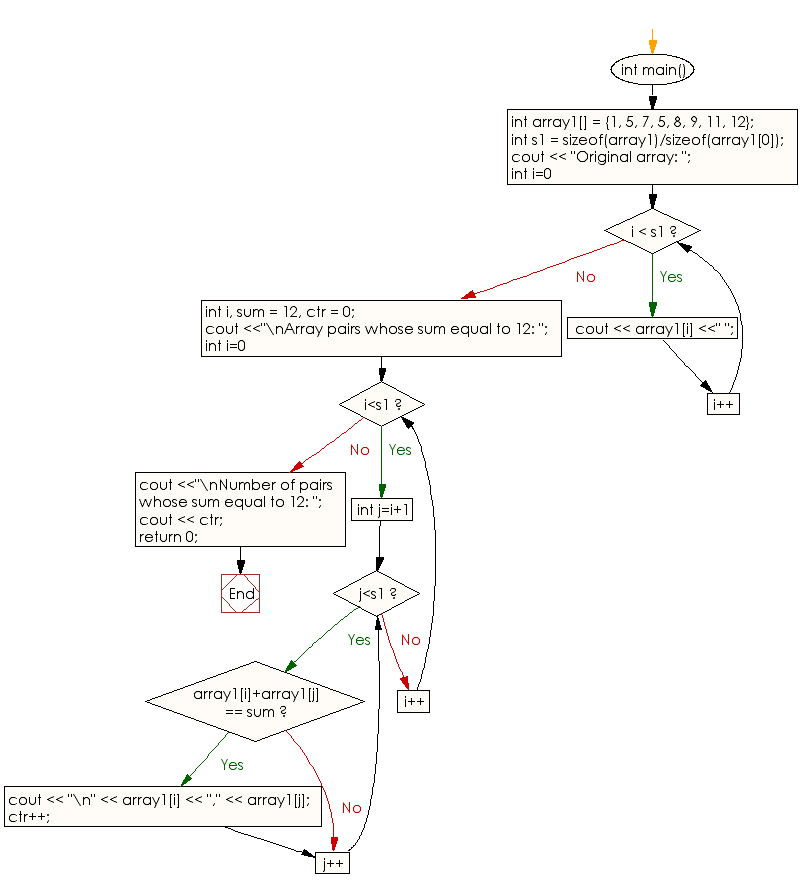﻿ C++ Exercises: Find the number of pairs of integers in a given array of integers whose sum is equal to a specified number - w3resourceC++ Exercises: Find the number of pairs of integers in a given array of integers whose sum is equal to a specified number

C++ Array: Exercise-27 with Solution

Write a C++ program to find the number of pairs of integers in a given array of integers whose sum is equal to a specified number.

Sample Solution:

C++ Code :

#include <iostream>
using namespace std;
int main()
{
int array1[] = {1, 5, 7, 5, 8, 9, 11, 12};
int s1 = sizeof(array1)/sizeof(array1);

cout << "Original array: ";

for (int i=0; i < s1; i++)
cout << array1[i] <<" ";

int i, sum = 12, ctr = 0;
cout <<"\nArray pairs whose sum equal to 12: ";

for (int i=0; i<s1; i++)
for (int j=i+1; j<s1; j++)
if (array1[i]+array1[j] == sum)
{
cout << "\n" << array1[i] << "," << array1[j];
ctr++;
}

cout <<"\nNumber of pairs whose sum equal to 12: ";
cout << ctr;

return 0;
}

Sample Output:

Original array: 1 5 7 5 8 9 11 12
Array pairs whose sum equal to 12:
1,11
5,7
7,5
Number of pairs whose sum equal to 12: 3

Flowchart:C++ Code Editor: